# Technical writing: using math

In a previous post I mentioned some general aspects of technical writing. In this one, I would like to talk about including mathematical expressions in technical documents.

There are two main ways to include math in your documents:

• using text; and

• using a graphic interface.

Using a graphic interface, such as the equation editor in LibreOffice Writer or MS Word, or MathType is convenient. You don't need to memorize anything and you can look at your expressions while creating them. Nevertheless, it can be slow compared to using text input — once you are comfortable with the syntax.

There are two main flavors of equations used over the internet:

• MathML is a W3C standard for equations and it is included in HTML5, so it would work in all modern browsers. The problem with it is that it is not designed to be written by hand. So one can use it if have some automatic way of generating the code.

• LaTeX is my suggested way to write equations. The learning curve might be a little bit steep at the beginning but it pays off.

One tool that helps with equations is MathPix Snip that automatically generates LaTeX or MathML code from an image, even a handwritten one. Another tool that is really useful is Detexify that let you draw a symbol and gives you the LaTeX syntax for it.

In the remaining of the posts I will show my suggestions for working with equations in LibreOffice and MS Word. If you are using LaTeX or MarkDown/ReStructuredText for your documents you are already using LaTeX for your equations.

## LibreOffice

LibreOffice has its own math editor with its own syntax and it works fine for small expressions, but it gets complicated for large equations or long algebraic manipulations. For LibreOffice I would suggest to use TexMaths, it is simple to use and works for the word processor (Writer) and presentations (Impress). I suppose it also works for spreadsheets (Calc), but I don't remember using equations in one.

## MS Office

MS Office has its own math editor as well, it works fine and is easy to use. Nevertheless, the same problem appears when you want long expressions. One option is to directly use LaTeX in Office but I prefer to use IguanaTex. It is a complement that allows to input equations similarly to TexMaths in LibreOffice.

You could also directly paste MathML equations into MS Word (>2013 and Windows).

## Use a SymPy

Indepent of the tool that you use to write your documents I strongly suggest to use a CAS (Computer Algebra System), such as Mathematica or SymPy. These programs have automatic generation of LaTeX and MathML from expression and that can ease the process a lot.

Let's check an example. Suppose that we have the function

\begin{equation*} f(x) = \exp(-x^2) \sin(3*x) \end{equation*}

and we want to compute its second derivative

\begin{equation*} f''(x) = \left(- 12 x \cos{\left(3 x \right)} + 2 \left(2 x^{2} - 1\right) \sin{\left(3 x \right)} - 9 \sin{\left(3 x \right)}\right) e^{- x^{2}} \end{equation*}

The following code gives us the LaTex code

from sympy import *
init_session()
f = exp(-x**2)*sin(3*x)
fxx = diff(f, x, 2)
print(latex(fxx))


that is

\left(- 12 x \cos{\left(3 x \right)} + 2 \left(2 x^{2} - 1\right) \sin{\left(3 x \right)} - 9 \sin{\left(3 x \right)}\right) e^{- x^{2}}


That corresponds to the code that I used above to render the equation

If, we wanted the MathML code of that expression we could use the following snippet

from sympy import *
init_session()
f = exp(-x**2)*sin(3*x)
fxx = diff(f, x, 2)
print(mathml(fxx, printer="presentation"))


notice the extra argument printer="presentation". If we want to add this to MS Word, for example, we could add the output (that I will not show because is really long) inside the following

<math xmlns = "http://www.w3.org/1998/Math/MathML">
[/itex]


When using Jupyter Notebook this can be done graphically with a right click over the expression. Then, the following menu is shown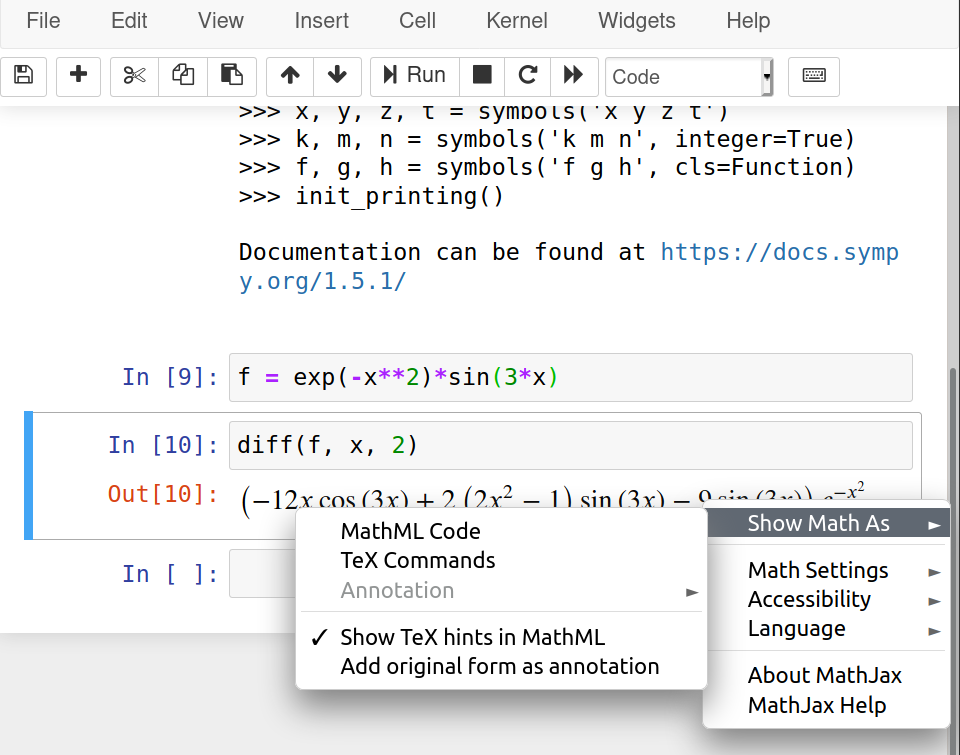This week a student asked me about downloading the videos from one of the courses from MS Stream. The problem is that if you are not a proprietary of the video you cannot download it. So, I will show you an option to download videos without being a proprietary of them.

## Prerequisites

You will need the following:

## destreamer installation

$git clone https://github.com/snobu/destreamer$ cd destreamer
$npm install$ npm run build


in a terminal.

If you do not want to play with environment variables, I suggest that you just add ffmpeg to the same folder as destreamer.

After that, you need to navigate to the folder where you downloaded destreamer and

$./destreamer.sh -i "https://web.microsoftstream.com/video/VIDEO_ID"  in Mac or Linux, $ destreamer.cmd -i "https://web.microsoftstream.com/video/VIDEO_ID"


in the command prompt in Windows, and

$destreamer.ps1 -i "https://web.microsoftstream.com/video/VIDEO_ID"  in PowerShell.VIDEO_ID refers to the identifier in MS Stream. If you want to download several files (like a complete course), you can create a file with the URLs and use $ destreamer.cmd -f filename.txt


# Randomized Marking of a Tetrahedron

Yesterday (June 4, 2020), Christian Howard posted on Twitter the following question

You are given a tetrahedron τ. For each triangular facet of τ, we uniformly at random mark one of their edges. What is the probability that there exists an edge of τ that is marked twice?

I thought about a little bit but I couldn't find how to count properly. Then, a number popped up in my mind out of the blue: $2/3$, but I don't know why. So, I decided to run a simulation to check this number.

The right answer is $51/81$ that is approximately 63%. This calculation is well explained in Christian's blog and with some cool drawings (and memes).

## The algorithm

The algorithm is quite simple. I number the edges in each face following a counter-clockwise orientation:

• face 0: edge 0, edge 1, edge 2

• face 1: edge 0, edge 3, edge 4

• face 2: edge 1, edge 5, edge 3

• face 3: edge 2, edge 4, edge 5

Then, I take each face and pick a random number from $(0, 1, 2)$ and mark the corresponding edge, and move to the following face. I repeat this process several times and I count the favorable cases and divide them by the number of trials to get an estimate of the probability.

Following is a Python code that follows this idea.

import numpy as np
import matplotlib.pyplot as plt

faces = np.array([
[0, 1, 2],
[0, 3, 4],
[1, 5, 3],
[2, 4, 5]])

def mark_edges():
marked_edges = np.zeros((6), dtype=int)
for face in faces:
num = np.random.randint(0, 3)
edge = face[num]
marked_edges[edge] += 1
return marked_edges

def comp_probs(N_min=1, N_max=5, ntrials=100):
prob = []
N_vals = np.logspace(N_min, N_max, ntrials, dtype=int)
for N in N_vals:
cont_marked = 0
for cont in range(N):
marked = mark_edges()
if 2 in marked:
cont_marked += 1
prob.append(cont_marked/N)
return N_vals, prob

#%% Computation
N_min = 1
N_max = 5
ntrials = 100
np.random.seed(seed=2)
N_vals, prob = comp_probs(N_min, N_max, ntrials)

#%% Plotting
plt.figure(figsize=(4, 3))
plt.hlines(0.63, 10**N_min, 10**N_max, color="#3f3f3f")
plt.semilogx(N_vals, np.array(prob), "o", alpha=0.5)
plt.xlabel("Number of trials")
plt.ylabel("Estimated probability")
plt.savefig("prob_tet.svg", dpi=300, bbox_inches="tight")
plt.show()


And we can see the following evolution for different number of trials.# Technical writing

This is the first post about technical writing * from a series that I will be creating during the course of this year. Technical writing is something that most of us have to deal with in different contexts. For example, in college coursework, research publications, software documentation. The main idea of the series is to mention some of the tricks that I have learned over the years and some tools that might come in handy.

Future posts will (probably) be about:

• Using figures;

• Using tables; and

• Managing bibliographic references.

## The current post

As mentioned above, technical writing is something that a lot of persons have to deal with. This is a skill that is sometimes overlooked, but it should not. According to the U.S. Bureau of Labor Statistics

Technical writers prepare instruction manuals, how-to guides, journal articles, and other supporting documents to communicate complex and technical information more easily.

And it is a desired skill in the workplace. Its demand is expected to grow around 10% in the current decade.

### Typography

The first thing that I should mention is that writing documents is typography. "Putting documents" together is typography because we are designing with text (Butterick, 2019). So, we should consider ourselves typographers since we are constantly designing documents.

I would suggest taking a look at "Butterick's Practical Typography" since it is a really good book about it and it reads smoothly. I will mention some important points here according to Butterick's "Typography in ten minutes":

1. The most important typographic selection is on the body text. This is due to the fact that it is most of the document.

2. Choose a point size between 10-12 points for printed documents and 15-25 pixels for digital documents.

3. Line spacing should be between 120-145 % of the point size.

4. Line length should be between 45-90 characters. This is roughly 2 or 3 small caps alphabets:

abcdefghijklmnopqrstuvwxyzabcdefghijklmnopqrstuvwxyzabcd

5. Mind the selection of your font. Try to avoid default fonts such as Arial, Calibri or Times New Roman.

### Editors

Another point that I want to touch in this post is about editors. The first question that arises is "what editor should I use?". The short answer is: use whatever your peers are using. That's my best advice; that way you have people to discuss with you about your doubts.

The long answer … is that each editor has its weak and strong points. I have written scientific papers in LaTeX, LibreOffice Writer and MS Word; all of them look professional. So, in the end, you can write your documents in several ways and achieve a similar result. I prefer to use LaTeX for long documents since it is centered in the structure of the document instead of the appearance and this is the way one should manage a long document like a dissertation, in my opinion.

If you just want me to pick one editor and suggest it to you, I would say that you should ride with LibreOffice. A good reference for it is "Designing with LibreOffice". Once you learn how to use styles you will ask how have you been writing documents all this time.

There are two main flavors for editors that I am going to discuss: WYSIWYG (What You See Is What You Get) and markup-based editors.

• WYSIWYG. This category is the one that most people is familiar with. Two examples are:

• LibreOffice writer; and

• Microsoft Word.

• Markup-based editors rely on marks on the "text" to differentiate sections and styles. In this case, your text looks like code, as seen in the following image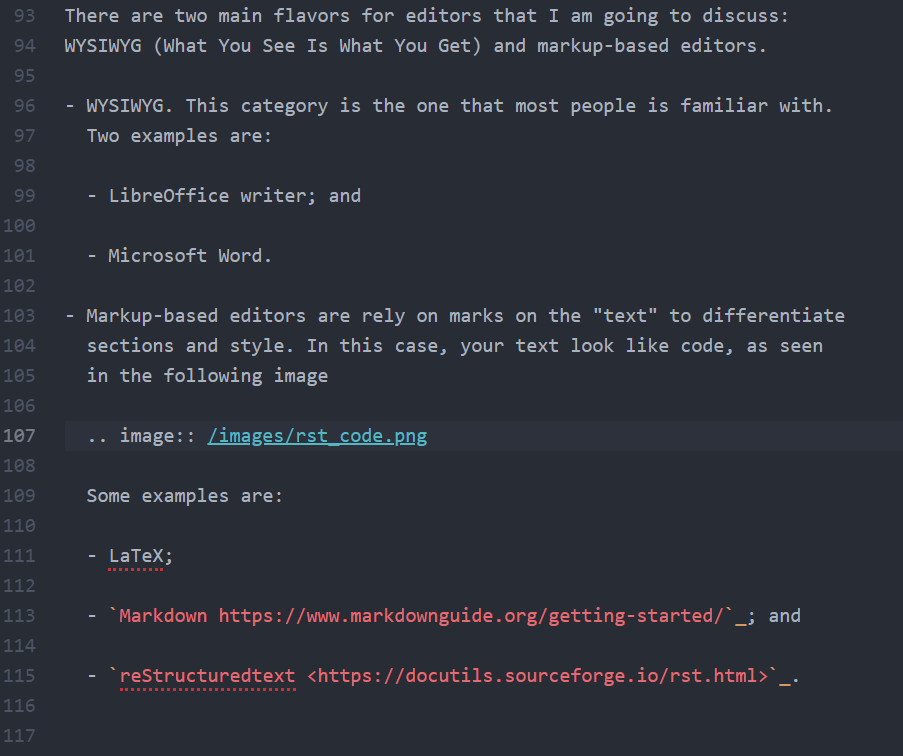Some examples are:

Independently of what your main editor is I suggest that you use Pandoc. It allows you to convert between several formats, making the process a little bit easier. There is even an editor based completely on it named Panwriter.

### References

1. Matthew Butterick (2019). Butterick's Practical Typography. Second edition, Matthew Butterick.

2. Wikibooks contributors. (2020). LaTeX. Wikibooks, The Free Textbook Project.

3. Bruce Byfield (2016). Designing with LibreOffice. Friends of OpenDocument, Inc.

4. Deville, S. (2015). Writing academic papers in plain text with Markdown and Jupyter notebook. Sylvain Deville.

*

This post is (somewhat) related to a previous post where I discussed research tools that most of us need but are not commonly taught in a formal fashion.

# Revisión ortográfica en Jupyter Notebook

El objetivo de esta publicación es mostrar cómo tener revisión automática de ortografía en Jupyter Notebook * para español, como se muestra a continuación.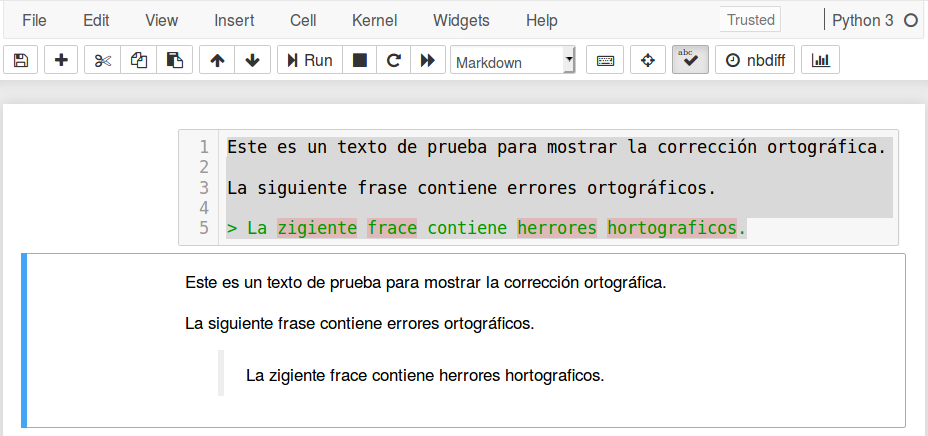Existen varias formas de realizar esto. Sin embargo, la forma más fácil es a través del complemento (nbextension) Spellchecker.

## Paso a paso

Los pasos a seguir son los siguientes:

1. Instalar Jupyter notebook extensions (nbextensions). Este incluye Spellchecker.

2. Ubicar los diccionarios en la carpeta donde está el complemento. Los diccionarios deben usar la codificación UTF-8.

3. Configurar la ruta de los diccionarios. Esta puede ser una URL o una ruta relativa respecto a la carpeta en donde se encuentra el complemento.

A continuación describiremos en detalle cada paso.

### Paso 1: Instalación de nbextensions

Existe una lista de complementos que agregan algunas funcionalidades comúnmente usadas a Jupyter notebook.

Escriba lo siguiente en una terminal, para instalarlo usando PIP.

pip install jupyter_contrib_nbextensions


Sin embargo, si se está usando Anaconda el método recomendado es usar conda, como se muestra a continuación.

conda install -c conda-forge jupyter_contrib_nbextensions


Esto debe instalar los complementos y también la interfaz de configuración. En el menú principal de Jupyter notebook aparecerá una nueva pestaña nombrada Nbextensions en donde se pueden elegir los complementos a usar. La apariencia es la siguiente.• Collapsible Headings: que permite ocultar secciones de los documentos.

• RISE: que convierte los notebooks en presentaciones.

### Paso 2: Diccionarios para español

La documentación de Spellchecker sugiere usar un script de Python para descargar diccionarios del proyecto Chromium. Sin embargo, estos tienen como codificación ISO-8859-1 (occidente) y falla para caracteres con tildes o virgulillas. Para que no haya problemas el diccionario debe tener codificación UTF-8. Pueden descargarse en este enlace.

Una vez que se tienen los diccionarios se deben ubicar en la ruta del complemento. En mi computador esta sería

~/.local/share/jupyter/nbextensions/spellchecker/


y dentro de esta los ubicaremos en

~/.local/share/jupyter/nbextensions/spellchecker/typo/dictionaries


Esta ubicación es arbitraria, lo importante es que necesitamos conocer la ruta relativa al complemento.

### Paso 3: Configuración complementos

Ahora, en la pestaña Nbextensions seleccionamos el complemento y llenamos los campos con la información de nuestro diccionario:

• language code to use with typo.js: es_ES

• url for the dictionary .dic file to use: ./typo/dictionaries/es_ES.dic

• url for the dictionary .aff file to use: ./typo/dictionaries/es_ES.aff

Esto se muestra a continuación.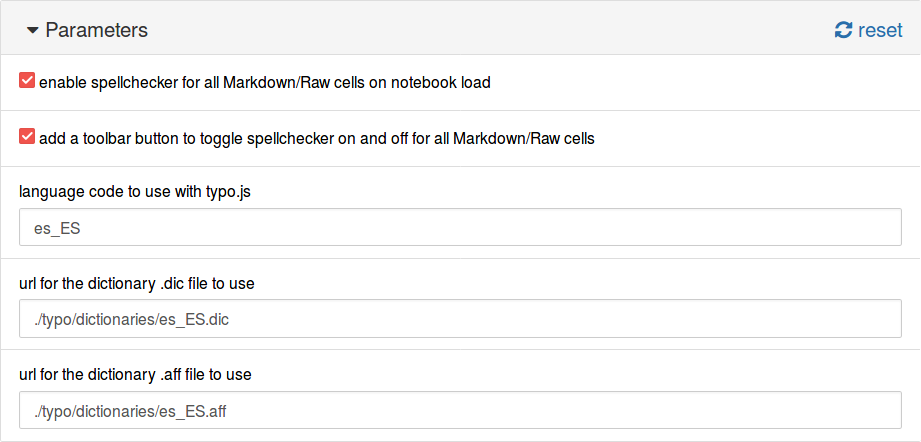Otra opción es usar la URL para los archivos. En https://github.com/wooorm/dictionaries están disponibles los diccionarios del proyecto hunspell en UTF-8. En este caso, la configuración sería:

• language code to use with typo.js: es_ES

• url for the dictionary .dic file to use: https://raw.githubusercontent.com/wooorm/dictionaries/master/dictionaries/es/index.dic

• url for the dictionary .aff file to use: https://raw.githubusercontent.com/wooorm/dictionaries/master/dictionaries/es/index.aff

Y se muestra a continuación.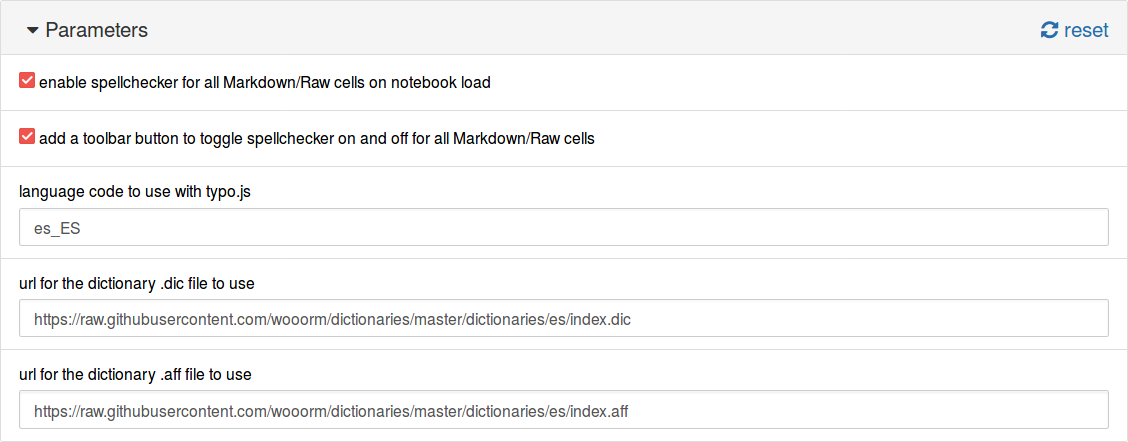*

Dado el público objetivo de esta publicación está hecha en español y no en inglés como se acostumbra en este blog.

# #SWDchallenge: Graph makeover

At storytelling with data, they run a monthly challenge on data visualization named #SWDchallenge. The main idea of the challenges is to test data visualization and storytelling skills.

Each month the challenge has a different topic. This month the challenge was to improve an existing graph. I decided to take a previous graph of mine that was published on a paper that was published on 2015.

## The "original"

The following is the graph that we are going to start with.This is not the exact same graph that was presented in the article on 2015, but it serves the purpose of the challenge. The data can be downloaded from here. This graph present the fraction of energy ($\eta$) transmitted for helical composites with different geometrical parameters. The parameters varied were:

• Pitch angle $\alpha$: the angle between consecutive layers;

• Composite thickness $D$, that is normalized to the wavelength $\lambda$; and

• Number of layers $N$ in each cell of the composite.

The following schematic illustrate these parameters.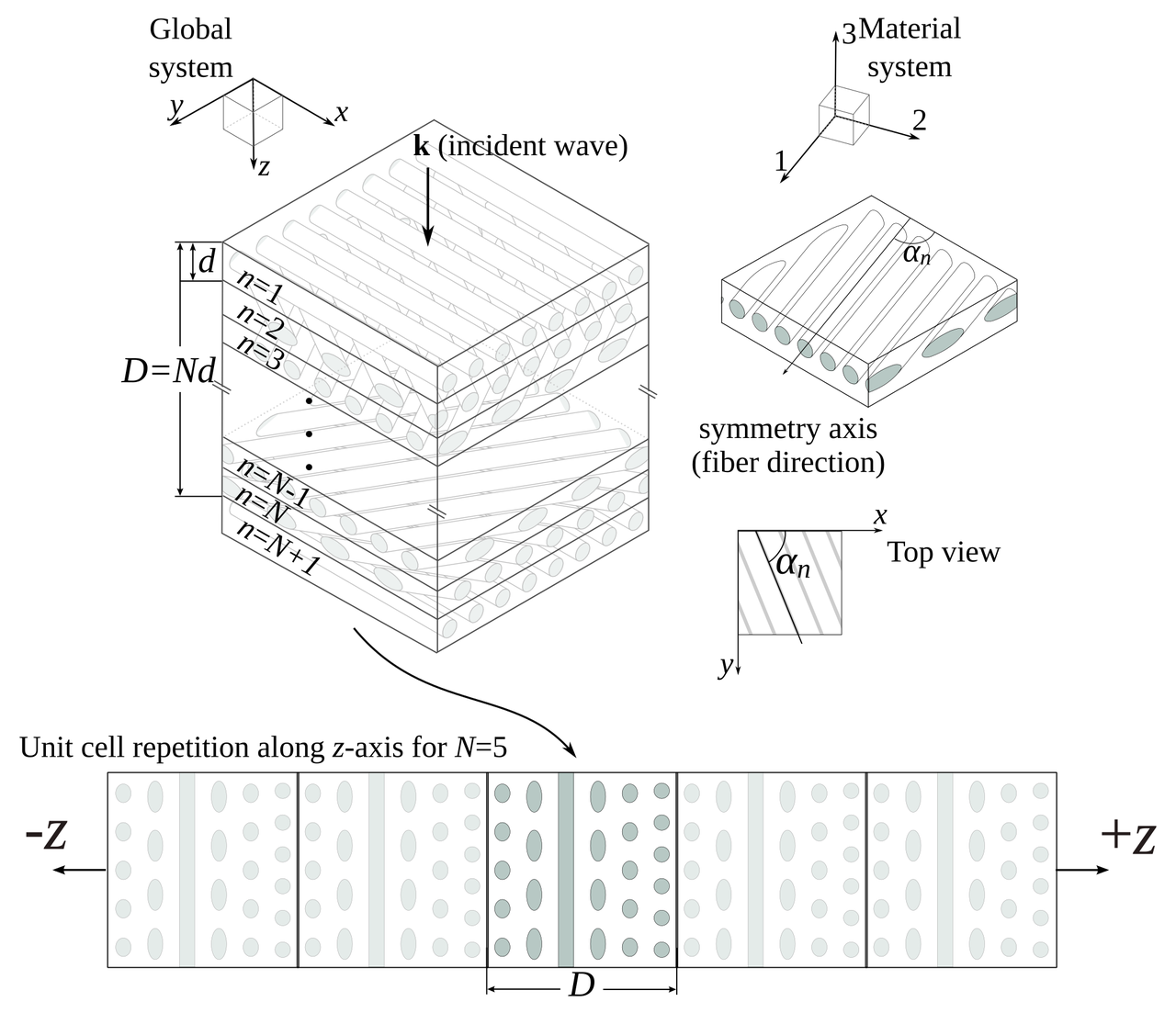I would not say that the graph is awful, and, in comparison to what you would find in most scientific literature it is even good. But … in the land of the blind, the one-eyed is king. So, let's enumerate what are the sins of the plot and see if we can correct them:

• It has two x axes.

• The legend is enclosed in a box that seems unnecessary.

• Right and top spines are not contributing to the plot.

• Annotations are stealing protagonism from the data.

• It looks clutterd with lines and markers.

• It is a spaghetti graph.

The following snippet generates this graph.

import numpy as np
from matplotlib import pyplot as plt
from matplotlib import rcParams

rcParams['font.family'] = 'serif'
rcParams['font.size'] = 16
rcParams['legend.fontsize'] = 15
rcParams['mathtext.fontset'] = 'cm'

markers = ['o', '^', 'v', 's', '<', '>', 'd', '*', 'x', 'D', '+', 'H']
usecols=range(1, 9))
labels = labels[0, :]

fig = plt.figure()
ax = plt.subplot(111)
for cont in range(8):
plt.plot(data[:, 0], data[:, cont + 1], marker=markers[cont],
label=r"$D/\lambda={:.3g}$".format(labels[cont]))

# First x-axis
xticks, xlabels = plt.xticks()
plt.xlabel(r"Number of layers - $N$", size=15)
plt.ylabel(r"Fraction of Energy - $\eta$", size=15)
ax.legend(loc='center left', bbox_to_anchor=(1, 0.5))

# Second x-axis
ax2 = ax.twiny()
ax2.set_xticks(xticks[2:])
ax2.set_xticklabels(180./xticks[2:])
plt.xlabel(r"Angle - $\alpha\ (^\circ)$", size=15)

plt.tight_layout()
plt.savefig("energy_vs_D_orig.svg")
plt.savefig("energy_vs_D_orig.png", dpi=300)


## Corrections

I will vindicate the graph one sin at a time, let's see how it turns out.

### It has two x axes

I, originally, added two axes to show both the number of layers and the angle between them at the same time. The general recommendation is to avoid this, so let's get rid of the top one.### Legend in a box

Pretty straightforward …### Right and top spines

Let's remove them### Annotations are stealing protagonism

Let's move all the annotations to the background by changing the color to a lighter gray.### Clutterd with lines and markers

Let's just keep the lines.And increase the linewidth.### It is a spaghetti graph

I think that a good option for this graph would be to highlight one line at a time. Doing this, we end up with.The following snippet generates this version.

import numpy as np
from matplotlib import pyplot as plt
from matplotlib import rcParams

# Plots setup
gray = '#757575'
plt.rcParams["mathtext.fontset"] = "cm"
plt.rcParams["text.color"] = gray
plt.rcParams["xtick.color"] = gray
plt.rcParams["ytick.color"] = gray
plt.rcParams["axes.labelcolor"] = gray
plt.rcParams["axes.edgecolor"] = gray
plt.rcParams["axes.spines.right"] = False
plt.rcParams["axes.spines.top"] = False
rcParams['font.family'] = 'serif'
rcParams['mathtext.fontset'] = 'cm'

def line_plots(data, highlight_line, title):
plt.title(title)
for cont, datum in enumerate(data[:, 1:].T):
if cont == highlight_line:
plt.plot(data[:, 0], datum, zorder=3, color="#984ea3",
linewidth=2)
else:
plt.plot(data[:, 0], datum, zorder=2, color=gray,
linewidth=1, alpha=0.5)

usecols=range(1, 9))
labels = labels[0, :]

plt.close("all")
plt.figure(figsize=(8, 4))
for cont in range(8):
ax = plt.subplot(2, 4, cont + 1)
title = r"$D/\lambda={:.3g}$".format(labels[cont])
line_plots(data, cont, title)
plt.ylim(0.4, 1.0)
if cont < 4:
plt.xlabel("")
ax.xaxis.set_ticks([])
ax.spines["bottom"].set_color("none")
else:
plt.xlabel(r"Number of layers - $N$")
if cont % 4 > 0:
ax.yaxis.set_ticks([])
ax.spines["left"].set_color("none")
else:
plt.ylabel(r"Fraction of Energy - $\eta$")

plt.tight_layout()
plt.savefig("energy_vs_D_7.svg")
plt.savefig("energy_vs_D_7.png", dpi=300)


## Final tweaks

Using Inkscape I added some final details to get the following version.# Isometric graphics in Inkscape: Part 2

Last week I posted a quick guide on isometric drawing using Inkscape. In that post, I showed how to obtain images that are projected to the faces of an isometric box.

After my post, I was asked by Biswajit Banerjee on Twitter if I could repeat the process with a more complex example, and he suggested the following schematicwhich, I guess, was created in Inkscape using the "Create 3D Box" option.

In this post, I will:

1. Use the same approach from last week to recreate this schematic

2. Suggest my preferred approach for drawing this schematic

## First approach

I will repeat the cheatsheet from last week. Keep in mind that Inkscape treats clockwise rotation as positive. Opposite to common notation in mathematics.Then, to create a box with desired dimensions we first create each rectangle with the right dimensiones (in parallel projections). In the following example we used faces with aspect ratios 3:2, 2:1 and 4:3. The box at the right is the figure obtained after applying the transformations described in the previous schematic.We can now proceed, to recreate the desired figure. I don't know the exact dimensions of the box; my guess is that the cross-section was a square and the aspect ratio was 5:1. After applying the transformations to each rectangle we obtain the following (adding some tweaks here and there).## Second approach

For this type of schematic, I would prefer to create an axonometric grid (File > Document Properties > Grids). After adding the grid to our canvas it is pretty straightforward to draw the figures in isometric view. The canvas should look similar to the following image.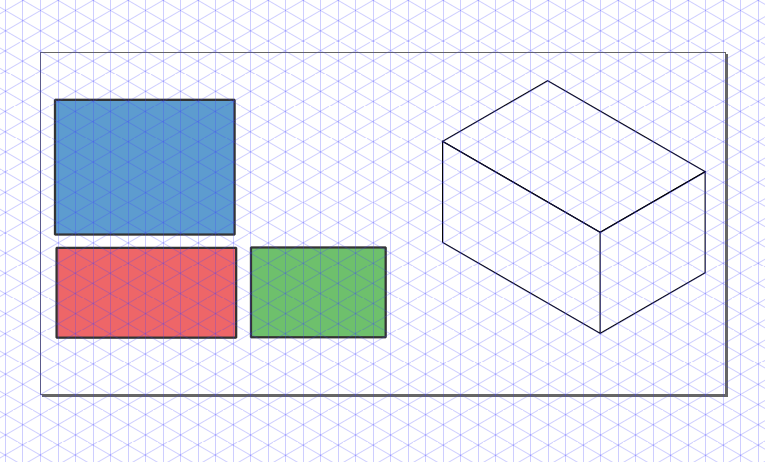We can then draw each face using the grid. If we want to be more precise we can activate Snap to Cusp Nodes. The following animation shows the step by step construction.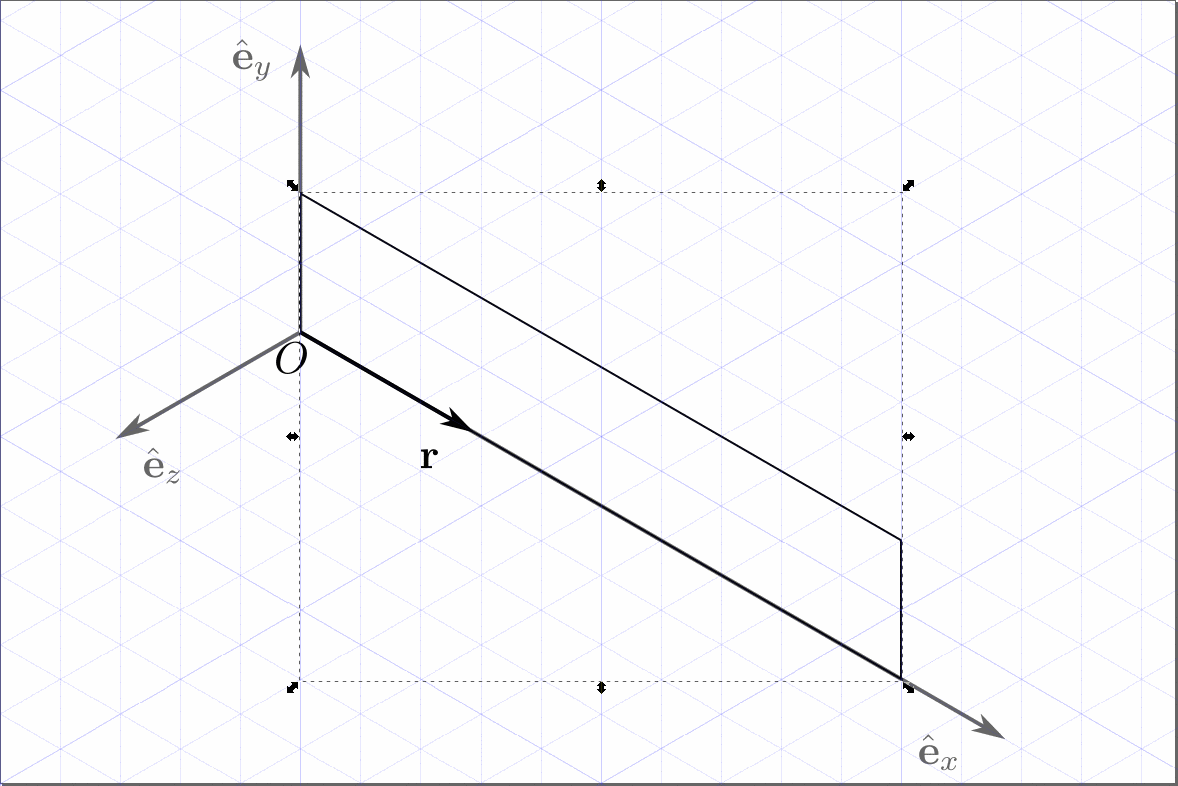And we obtain the final image.## Conclusion

As I mentioned, Inkscape can be used for drawing simple figures in isometric projection. Nevertheless, I strongly suggest to use a CAD like FreeCAD for more complicated geometries.

# Isometric graphics in Inkscape

Sometimes I find myself in need of making a schematic using an isometric projection. When the schematic is complicated the best shot is to use some CAD like FreeCAD, but sometimes it's just needed in simple diagrams. Another situation where this is a common needed is in video games, where "isometric art" and pixel art are pretty common.

What we want is depicted in the following figure.That is, we want to start with some information that is drawn, or written in the case of the example, and we want to obtain how would it been seen on one of the faces of an isometric box.

Following, I will describe briefly the transformations involved in this process. If you are just interested in the recipe for doing this in Inkscape, skip to the end of this post.

## Transformations involved

Since we are working on a computer screen, we are talking of 2 dimensions. Hence, all transformations are represented by 2×2 matrices. In the particular example of interest in this post we need the following transformations:

1. Vertical scaling

2. Horizontal skew

3. Rotation

Following are the transformation matrices.

### Scaling in the vertical direction

The matrix is given by

\begin{equation*} M_\text{scaling} = \begin{bmatrix} 1 &0\\ 0 &a\end{bmatrix}\, , \end{equation*}

where $a$ is the scaling factor.

### Horizontal skew

The matrix is given by

\begin{equation*} M_\text{skew} = \begin{bmatrix} 1 &\tan a\\ 0 &1\end{bmatrix}\, , \end{equation*}

where $a$ is the skewing angle.

### Rotation

The matrix is given by

\begin{equation*} M_\text{rotation} = \begin{bmatrix} \cos a &-\sin a\\ \sin a &\cos a\end{bmatrix}\, , \end{equation*}

where $a$ is the rotation angle.

### Complete transformation

The complete transformation is given by

\begin{equation*} M_\text{complete} = M_\text{rotation} M_\text{skew} M_\text{scaling}\, , \end{equation*}

particularly,

\begin{align*} &M_\text{side} = \frac{1}{2}\begin{bmatrix} \sqrt{3} & 0\\ -1 &2\end{bmatrix}\approx \begin{bmatrix} 0.866 & 0.000\\ -0.500 &1.000\end{bmatrix}\, , \\ &M_\text{front} = \frac{1}{2}\begin{bmatrix} \sqrt{3} & 0\\ 1 &2\end{bmatrix}\approx \begin{bmatrix} 0.866 & 0.000\\ 0.500 &1.000\end{bmatrix}\, , \\ &M_\text{plant} = \frac{1}{2}\begin{bmatrix} \sqrt{3} & -\sqrt{3}\\ -1 &1\end{bmatrix}\approx \begin{bmatrix} 0.866 & -0.866\\ 0.500 &0.500\end{bmatrix}\, . \end{align*}

The values seem a bit arbitrary, but they can be obtained from the isometric projection itself. But that explanation would be a bit too long for this post.

### Tranformation in Inkscape

We already have the transformation matrices and we can use them in Inkscape. But first, we need to understand how it works. Inkscape uses SVG, the web standard for vector graphics. Transformations in SVG are done using the following matrix

\begin{equation*} \begin{bmatrix} a &c &e\\ b &d &f\\ 0 &0 &1\end{bmatrix}\, , \end{equation*}

that uses homogeneous coordinates. So, one can just press Shift + Ctrl + M and type the components of the matrices obtained above for $a$, $b$, $c$, and $d$; leaving $e$ and $f$ as zero.

My preferred method, though, is to apply each transformation after the other in the Transform dialog (Shift + Ctrl + M). And, this is the method presented in the cheatsheet at the bottom of this post.

## TL;DR

If you are just interested in the transformations needed in Inkscape you can check the cheatsheet below. It uses third-angle as presented below.### Cheatsheet

Keep in mind that Inkscape treats clockwise rotation as positive. Opposite to common notation in mathematics.# Finite differences in infinite domains

## Finite differences in infinite domains

Because of my friend, Edward Villegas, I ended up thinking about using a change of variables when solving an eigenvalue problem with finite difference.

### The problem

Let's say that we want to solve a differential equation over an infinite domain. A common case is to solve the Time independent Schrödinger equation subject to a potential $V(x)$. For example

\begin{equation*} -\frac{1}{2}\frac{\mathrm{d}^2}{\mathrm{d}x^2}\psi(x) + V(x) \psi(x) = E\psi(x),\quad \forall x\in (-\infty, \infty), \end{equation*}

where we want to find the pairs of eigenvalues/eigenfunctions $(E_n, \psi_n(x))$.

What I normally do when using finite differences is to regularly divide the domain. Where I take a large enough domain, so the solution have decayed close to zero. What I do in this post is to make a change of variable to render the interval finite first and then regularly divide the transformed domain in finite intervals.

### My usual approach

My usual approach is to approach the second derivative with a centered difference for the point $x_i$ like this

\begin{equation*} \frac{\mathrm{d}^2 f(x)}{\mathrm{d}x^2} \approx \frac{f(x + \Delta x) - 2 f(x_i) + f(x - \Delta x)}{\Delta x^2}\, , \end{equation*}

with $\Delta x$ the separation between points.

We can solve this in Python with the following snippet:

import numpy as np
from scipy.sparse import diags
from scipy.sparse.linalg import eigs

def regular_FD(pot, npts=101, x_max=10, nvals=6):
"""
Find eigenvalues/eigenfunctions for Schrodinger
equation for the given potential pot using
finite differences
"""
x = np.linspace(-x_max, x_max, npts)
dx = x - x
D2 = diags([1, -2, 1], [-1, 0, 1], shape=(npts, npts))/dx**2
V = diags(pot(x))
H = -0.5*D2 + V
vals, vecs = eigs(H, k=nvals, which="SM")
return x, np.real(vals), vecs


Let's setup the plotting details.

# Jupyter notebook plotting setup & imports
%matplotlib notebook
import matplotlib.pyplot as plt

gray = '#757575'
plt.rcParams["figure.figsize"] = 6, 4
plt.rcParams["mathtext.fontset"] = "cm"
plt.rcParams["text.color"] = gray
fontsize = plt.rcParams["font.size"] = 12
plt.rcParams["xtick.color"] = gray
plt.rcParams["ytick.color"] = gray
plt.rcParams["axes.labelcolor"] = gray
plt.rcParams["axes.edgecolor"] = gray
plt.rcParams["axes.spines.right"] = False
plt.rcParams["axes.spines.top"] = False


Let's consider the Quantum harmonic oscillator, that has as eigenvalues

\begin{equation*} E_n = n + \frac{1}{2},\quad \forall n = 0, 1, 2 \cdots \end{equation*}

Using the finite difference method we have values that are really close to the analytic ones.

x, vals, vecs = regular_FD(lambda x: 0.5*x**2, npts=201)
vals


with output

array([0.4996873 , 1.49843574, 2.49593063, 3.49216962, 4.48715031,
5.4808703 ])

With the analytic ones

[0.5, 1.5, 2.5, 3.5, 4.5, 5.5])


If we plot these two, we obtain the following.

plt.figure()
plt.plot(anal_vals)
plt.plot(vals, "o")
plt.xlabel(r"$n$", fontsize=16)
plt.ylabel(r"$E_n$", fontsize=16)
plt.legend(["Analytic", "Finite differences"])
plt.tight_layout();Let's see the eigenfunctions.

plt.figure()
plt.plot(x, np.abs(vecs[:, :3])**2)
plt.xlim(-6, 6)
plt.xlabel(r"$x$", fontsize=16)
plt.ylabel(r"$|\psi_n(x)|^2$", fontsize=16)
plt.yticks([])
plt.tight_layout();One inconvenient with this method is the redundant sampling towards the extreme of the intervals, while under sample the middle part.

### Changing the domain

Let's now consider the case where we transform the infinite domain to a finite one with a change of variable

\begin{equation*} \xi = \xi(x) \end{equation*}

with $\xi \in (-1, 1)$. Two options for this transformation are:

• $\xi = \tanh x$; and

• $\xi = \frac{2}{\pi} \arctan x$.

Making this change of variable the equation we need to solve the following equation

\begin{equation*} -\frac{1}{2}\left(\frac{\mathrm{d}\xi}{\mathrm{d}x}\right)^2\frac{\mathrm{d}^2}{\mathrm{d}\xi^2}\psi(\xi) - \frac{1}{2}\frac{\mathrm{d}^2\xi}{\mathrm{d}x^2}\frac{\mathrm{d}}{\mathrm{d}\xi}\psi(\xi) + V(\xi) \psi(\xi) = E\psi(\xi) \end{equation*}

The following snippet solve the eigenproblem for the mapped domain:

def mapped_FD(pot, fun, dxdxi, dxdxi2, npts=101, nvals=6, xi_tol=1e-6):
"""
Find eigenvalues/eigenfunctions for Schrodinger
equation for the given potential pot using
finite differences over a mapped domain on (-1, 1)
"""
xi = np.linspace(-1 + xi_tol, 1 - xi_tol, npts)
x = fun(xi)
dxi = xi - xi
D2 = diags([1, -2, 1], [-1, 0, 1], shape=(npts, npts))/dxi**2
D1 = 0.5*diags([-1, 1], [-1, 1], shape=(npts, npts))/dxi
V = diags(pot(x))
fac1 = diags(dxdxi(xi)**2)
fac2 = diags(dxdxi2(xi))
H = -0.5*fac1.dot(D2) - 0.5*fac2.dot(D1) + V
vals, vecs = eigs(H, k=nvals, which="SM")
return x, np.real(vals), vecs


#### First transformation: $\xi = \tanh(x)$

Let's consider first the transformation

\begin{equation*} \xi = \tanh(x)\, . \end{equation*}

In this case

\begin{equation*} \frac{\mathrm{d}\xi}{\mathrm{d}x} = 1 - \tanh^2(x) = 1 - \xi^2\, , \end{equation*}

and

\begin{equation*} \frac{\mathrm{d}^2\xi}{\mathrm{d}x^2} = -2\tanh(x)[1 - \tanh^2(x)] = -2\xi[1 - \xi^2]\, . \end{equation*}

We need to define the functions

pot = lambda x: 0.5*x**2
fun = lambda xi: np.arctanh(xi)
dxdxi = lambda xi: 1 - xi**2
dxdxi2 = lambda xi: -2*xi*(1 - xi**2)


and run the function

x, vals, vecs = mapped_FD(pot, fun, dxdxi, dxdxi2, npts=201)
vals


And we obtain the following

array([0.49989989, 1.4984226 , 2.49003572, 3.46934257, 4.46935021,
5.59552989])

If we compare with the analytic values we get the following.

plt.figure()
plt.plot(anal_vals)
plt.plot(vals, "o")
plt.legend(["Analytic", "Finite differences"])
plt.xlabel(r"$n$", fontsize=16)
plt.ylabel(r"$E_n$", fontsize=16)
plt.tight_layout();And the following are the eigenfunctions.

plt.figure()
plt.plot(x, np.abs(vecs[:, :3])**2)
plt.xlim(-6, 6)
plt.xlabel(r"$x$", fontsize=16)
plt.ylabel(r"$|\psi_n(x)|^2$", fontsize=16)
plt.yticks([])
plt.tight_layout();#### Second transformation: $\xi = \frac{2}{\pi}\mathrm{atan}(x)$

Let's consider first the transformation

\begin{equation*} \xi = \frac{2}{\pi}\mathrm{atan}(x)\, . \end{equation*}

In this case

\begin{equation*} \frac{\mathrm{d}\xi}{\mathrm{d}x} = \frac{2}{\pi(1 + x^2)} = \frac{2 \cos^2\xi}{\pi} \, , \end{equation*}

and

\begin{equation*} \frac{\mathrm{d}^2\xi}{\mathrm{d}x^2} = -\frac{4x}{\pi(1 + x^2)^2} = -\frac{4 \cos^4\xi \tan \xi}{\pi}\, . \end{equation*}

Once, again, we define the functions.

pot = lambda x: 0.5*x**2
fun = lambda xi: np.tan(0.5*np.pi*xi)
dxdxi = lambda xi: 2/np.pi * np.cos(0.5*np.pi*xi)**2
dxdxi2 = lambda xi: -4/np.pi * np.cos(0.5*np.pi*xi)**4 * np.tan(0.5*np.pi*xi)


and run the function

x, vals, vecs = mapped_FD(pot, fun, dxdxi, dxdxi2, npts=201)
vals


to obtain the following eigenvalues

array([0.49997815, 1.49979632, 2.49930872, 3.49824697, 4.49627555,
5.49295665])

with this plot

plt.figure()
plt.plot(anal_vals)
plt.plot(vals, "o")
plt.legend(["Analytic", "Finite differences"])
plt.xlabel(r"$n$", fontsize=16)
plt.ylabel(r"$E_n$", fontsize=16)
plt.tight_layout();and the following eigenfunctions.

plt.figure()
plt.plot(x, np.abs(vecs[:, :3])**2)
plt.xlabel(r"$x$", fontsize=16)
plt.ylabel(r"$|\psi|^2$", fontsize=16)
plt.xlim(-6, 6)
plt.xlabel(r"$x$", fontsize=16)
plt.ylabel(r"$|\psi_n(x)|^2$", fontsize=16)
plt.yticks([])
plt.tight_layout();### Conclusion

The method works fine, although the differential equation is more convoluted due to the change of variable. Although there are more elegant methods to consider infinite domains, this is simple enough to be solved in 10 lines of code.

We can see that the mapping $\xi = \mathrm{atan}(x)$, covers better the domain than $\xi = \tanh(x)$, where most of the points are placed in the center of the interval.

This post was written in the Jupyter notebook. You can download this notebook, or see a static view on nbviewer.

# Cyclic colormaps comparison

I started this post looking for a diffusion map on Python, that I didn't find. Then I continued following an example on manifold learning by Jake Vanderplas on a different dataset. It worked nicely,but the colormap used is Spectral, that is divergent. This made me think about using a cyclic colormap, and ended up in this StackOverflow question. And I decided to compare some cyclic colormaps.

I picked up colormaps from different sources

• phase

• hue_L60

• erdc_iceFire

• nic_Edge

• colorwheel

• cyclic_mrybm_35_75_c68

• cyclic_mygbm_30_95_c78

and, of course, hsv. You can download the colormaps in text format from here.

## Comparison

For all the examples below the following imports are done:

from __future__ import division, print_function
import numpy as np
import matplotlib.pyplot as plt
from matplotlib.colors import LinearSegmentedColormap
from colorspacious import cspace_convert


### Color map test image

Peter Kovesi proposed a way to compare cyclic colormaps on a paper on 2015. It consists of a spiral ramp with an undulation. In polar coordinates it reads

\begin{equation*} c = (A \rho^p \sin(n \theta) + \theta)\, \mathrm{mod}\, 2\pi \end{equation*}

with $A$ the amplitude of the oscilation, $\rho$ the normalized radius in [0, 1], $p$ a positive number, and $n$ the number of cycles.

And the following function creates the grid in Python

def circle_sine_ramp(r_max=1, r_min=0.3, amp=np.pi/5, cycles=50,
power=2, nr=50, ntheta=1025):
r, t = np.mgrid[r_min:r_max:nr*1j, 0:2*np.pi:ntheta*1j]
r_norm = (r - r_min)/(r_max - r_min)
vals = amp * r_norm**power * np.sin(cycles*t) + t
vals = np.mod(vals, 2*np.pi)
return t, r, vals


The following is the result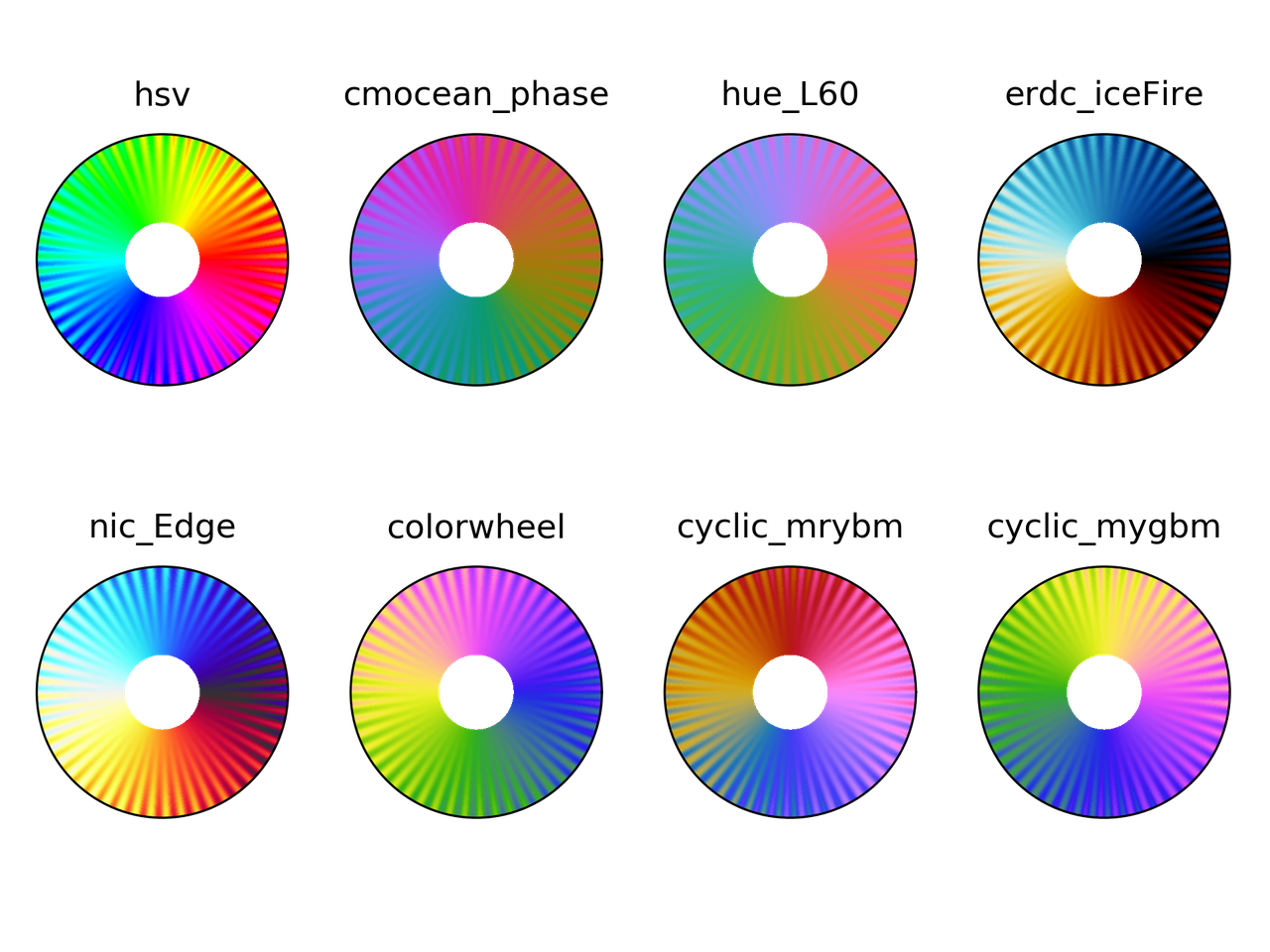### Colorblindness test

t, r, vals = circle_sine_ramp(cycles=0)
cmaps = ["hsv",
"cmocean_phase",
"hue_L60",
"erdc_iceFire",
"nic_Edge",
"colorwheel",
"cyclic_mrybm",
"cyclic_mygbm"]
severity = [0, 50, 50, 50]
for colormap in cmaps:
fig = plt.figure()
for cont in range(4):
cvd_space = {"name": "sRGB1+CVD",
"cvd_type": cvd_type[cont],
"severity": severity[cont]}
data2 = cspace_convert(data, cvd_space, "sRGB1")
data2 = np.clip(data2, 0, 1)
cmap = LinearSegmentedColormap.from_list('my_colormap', data2)
ax = plt.subplot(2, 2, 1 + cont, projection="polar")
ax.pcolormesh(t, r, vals, cmap=cmap)
ax.set_xticks([])
ax.set_yticks([])
plt.suptitle(colormap)
plt.tight_layout()
plt.savefig(colormap + ".png", dpi=300)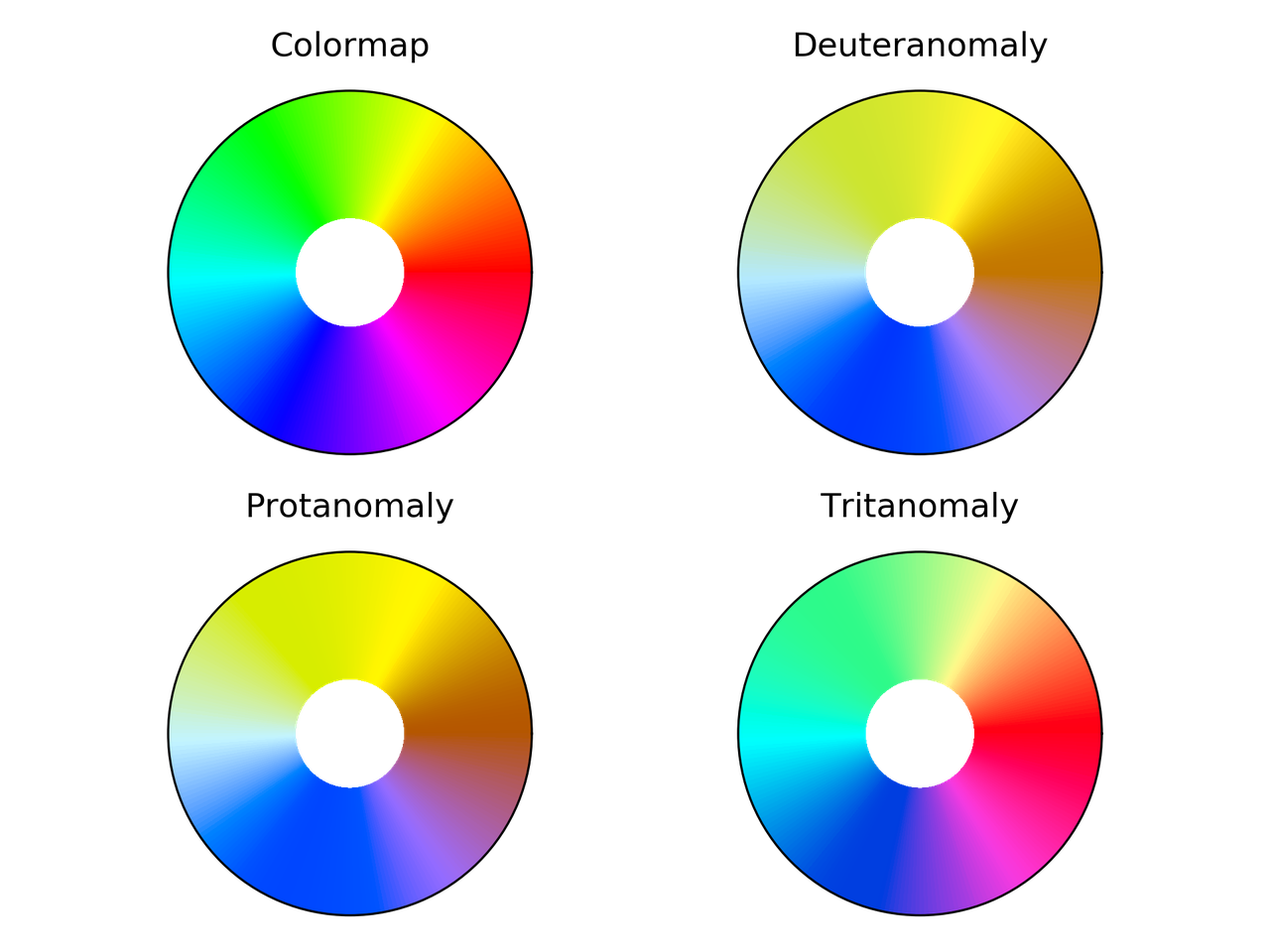hsv colormap comparison for different color vision deficiencies.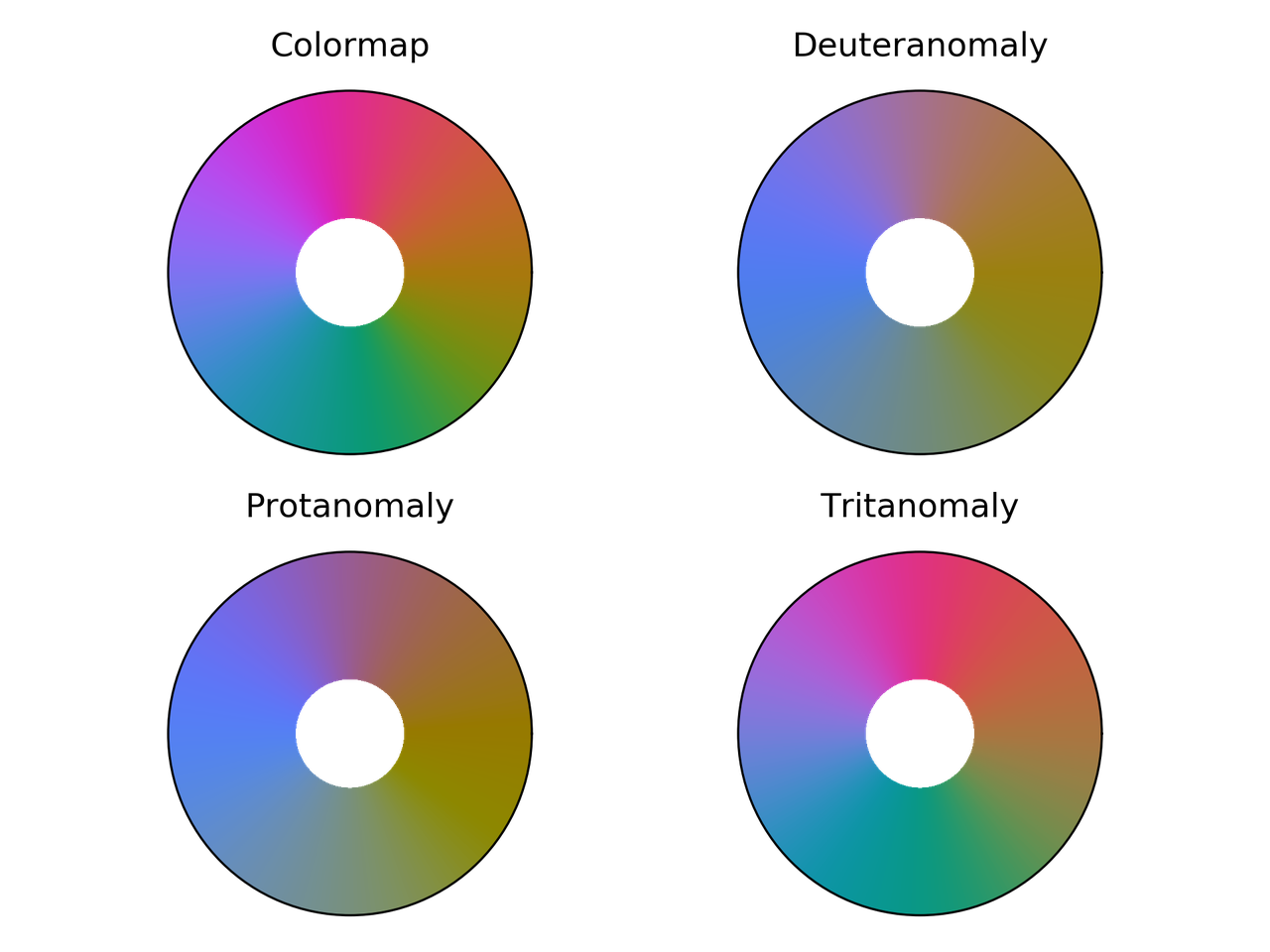Phase colormap comparison for different color vision deficiencies.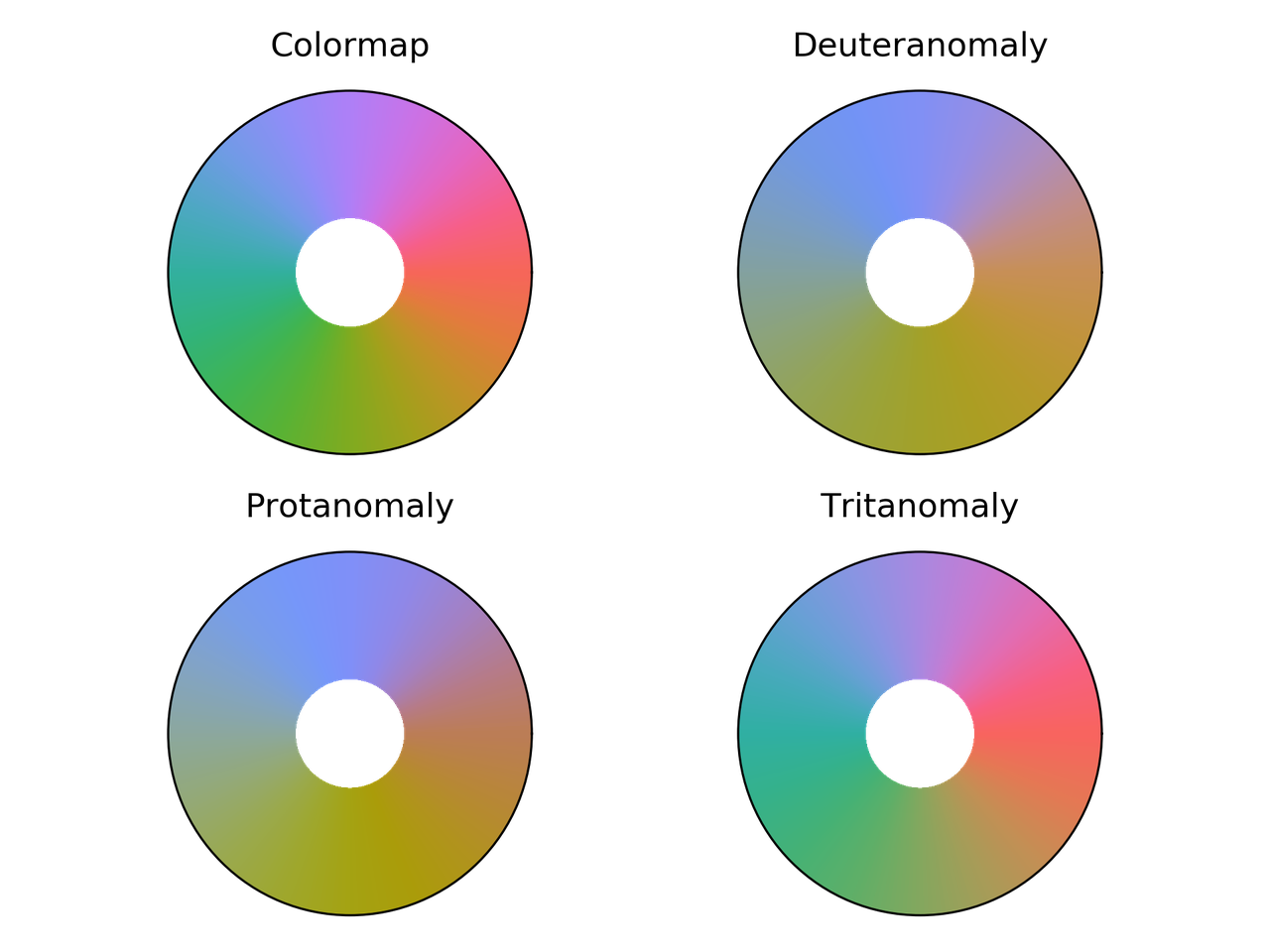hue_L60 colormap comparison for different color vision deficiencies.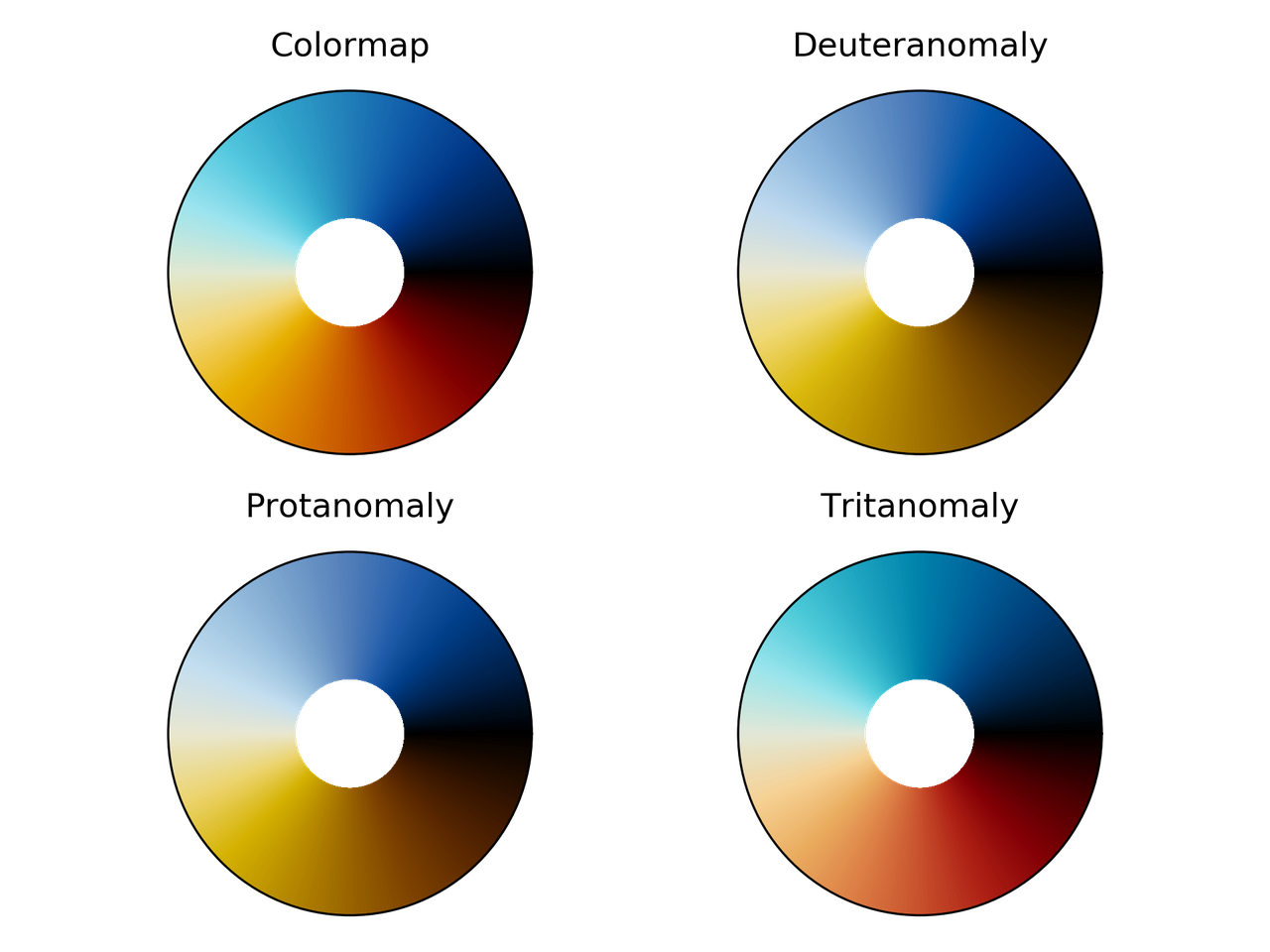erdc_iceFire colormap comparison for different color vision deficiencies.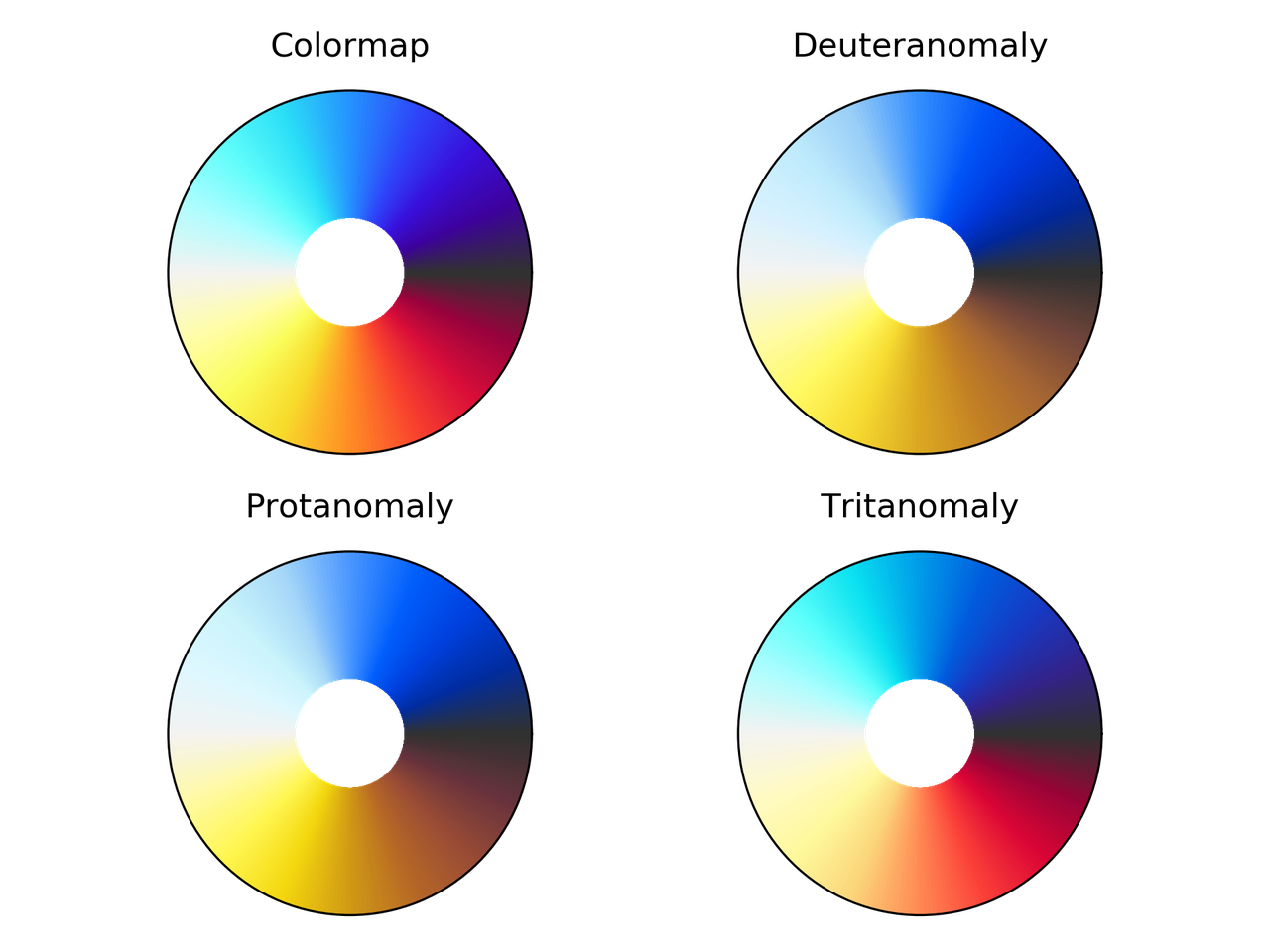nic_Edge colormap comparison for different color vision deficiencies.Colorwheel colormap comparison for different color vision deficiencies.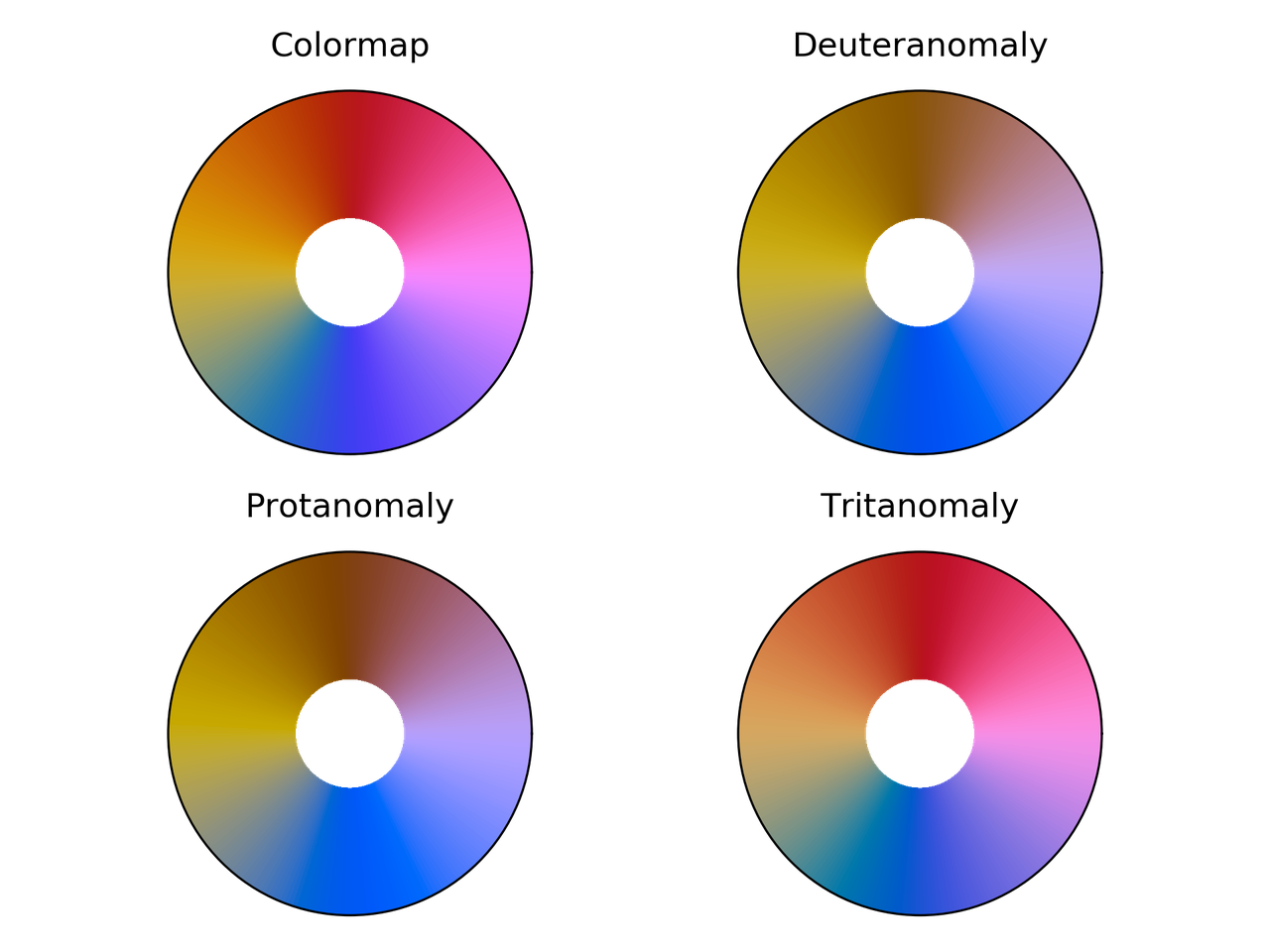Cyclic_mrybm colormap comparison for different color vision deficiencies.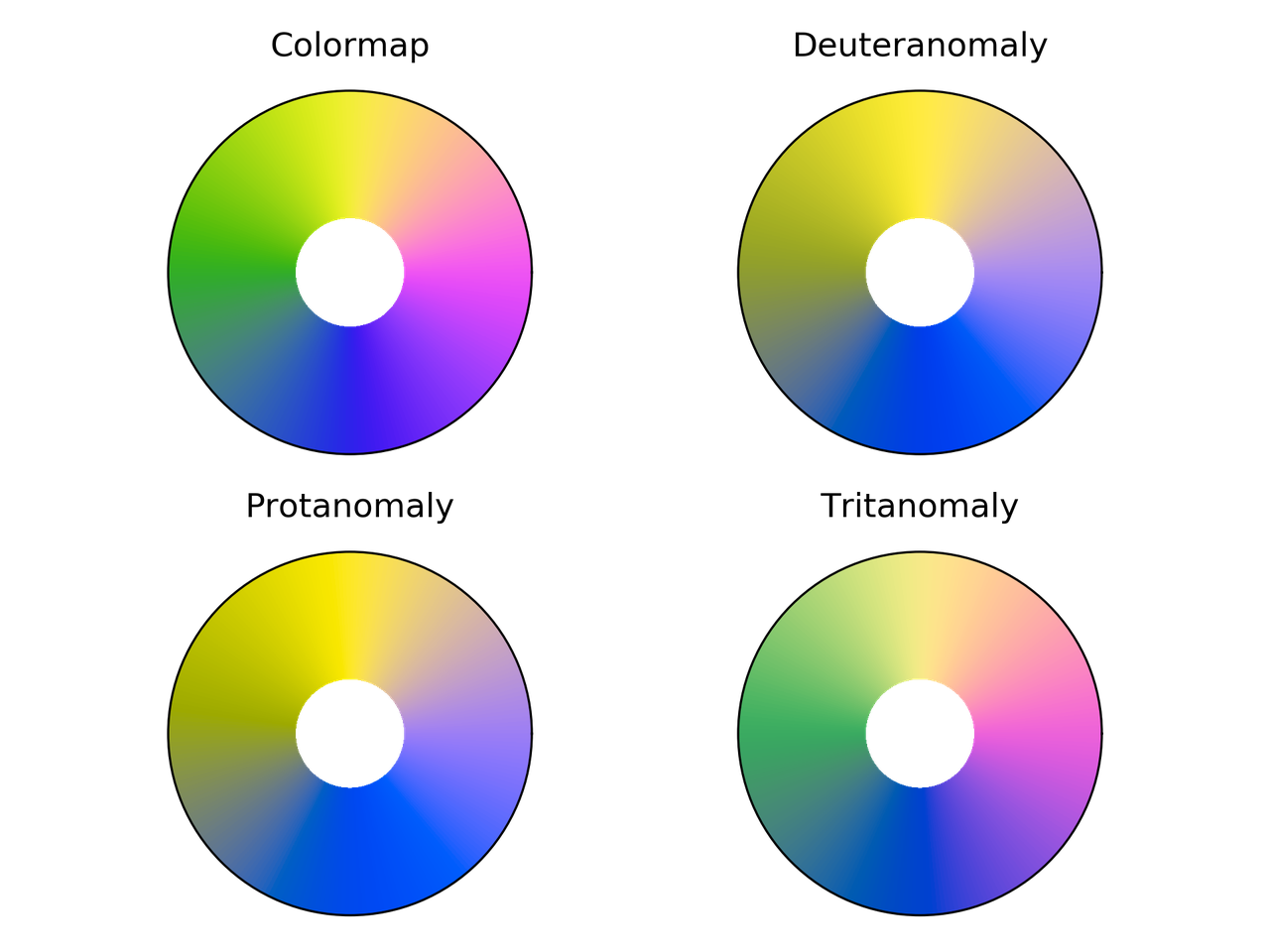Cyclic_mygbm colormap comparison for different color vision deficiencies.

## Randomly generated cyclic colormaps

What if we generate some random colormaps that are cyclic? How would they look like?

Following are the snippet and resulting colormaps.

from __future__ import division, print_function
import numpy as np
from mpl_toolkits.mplot3d import Axes3D
import matplotlib.pyplot as plt
from matplotlib.colors import LinearSegmentedColormap

# Next line to silence pyflakes. This import is needed.
Axes3D

plt.close("all")
fig = plt.figure()
fig2 = plt.figure()
nx = 4
ny = 4
azimuths = np.arange(0, 361, 1)
zeniths = np.arange(30, 70, 1)
values = azimuths * np.ones((30, 361))
for cont in range(nx * ny):
np.random.seed(seed=cont)
mat = np.random.rand(3, 3)
rot_mat, _ = np.linalg.qr(mat)
t = np.linspace(0, 2*np.pi, 256)
z = 0.0*np.cos(t)
X = np.vstack((x, y, z))
X = rot_mat.dot(X) + center

ax = fig.add_subplot(ny, nx, 1 + cont, projection='polar')
cmap = LinearSegmentedColormap.from_list('my_colormap', X.T)
ax.pcolormesh(azimuths*np.pi/180.0, zeniths, values, cmap=cmap)
ax.set_xticks([])
ax.set_yticks([])

ax2 = fig2.add_subplot(ny, nx, 1 + cont, projection='3d')
ax2.plot(X[0, :], X[1, :], X[2, :])
ax2.set_xlim(0, 1)
ax2.set_ylim(0, 1)
ax2.set_zlim(0, 1)
ax2.view_init(30, -60)
ax2.set_xticks([0, 0.5, 1.0])
ax2.set_yticks([0, 0.5, 1.0])
ax2.set_zticks([0, 0.5, 1.0])
ax2.set_xticklabels([])
ax2.set_yticklabels([])
ax2.set_zticklabels([])

fig.savefig("random_cmaps.png", dpi=300, transparent=True)
fig2.savefig("random_cmaps_traj.svg", transparent=True)16 randomly generated colormaps.Trajectories in RGB space for the randomly generated colormaps.

A good idea would be to take these colormaps and optimize some perceptual parameters such as lightness to get some usable ones.

## Conclusions

I probably would use phase, colorwheel, or mrybm in the future.Initial image using phase.Initial image using colorwheel.Initial image using mrybm.

## References

1. Peter Kovesi. Good Colour Maps: How to Design Them. arXiv:1509.03700 [cs.GR] 2015